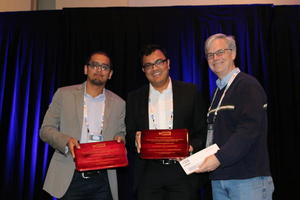# 2016

## V. Chandrasekaran and P. Shah win the 2016 INFORMS Optimization Society Young Researcher PrizeParikshit Shah (left), Venkat Chandrasekaran, and Andrew Schaefer.

## Citation

Venkat Chandrasekaran and Parikshit Shah are awarded the 2016 INFORMS Optimization Society Prize for Young Researchers for their paper, "Relative Entropy Relaxations for Signomial Optimization," SIAM J. Optimization 26 (2016), pp. 1147-1173. The paper presents a new solution approach for signomial programs, a class of important, yet difficult, nonconvex programs involving the minimization of a signomial subject to nonnegativity constraints on signomials, where a signomial equals the linear combination of exponentials of linear functions in the decision vector. Similar to semidefinite hierarchies for polynomial programs, the authors introduce a hierarchy of bounds for signomial programs based on decomposing signomials using so-called SAGE (sum-of-arithmetic-geometric-mean exponentials) functions, and the authors show how the bounds can then be computed efficiently (for low levels of the hierarcy) using convex relative-entropy optimization. They prove that the bounds converge to the optimal value of the signomial program under certain conditions and discuss multiple interesting properties of their approach. A particular highlight of the paper shows that, although a signomial program can be recast as a polynomial program, doing so introduces certain deficiences, to which the authors' direct approach is not susceptible.

## Selection committee

Sam Burer (chair), Jon Lee, Andrew Schaefer, Cole Smith